Скачать презентацию Estimating Distinct Elements Optimally David Woodruff IBM Based

0504226431f037f6e84efb8693823239.ppt

• Количество слайдов: 17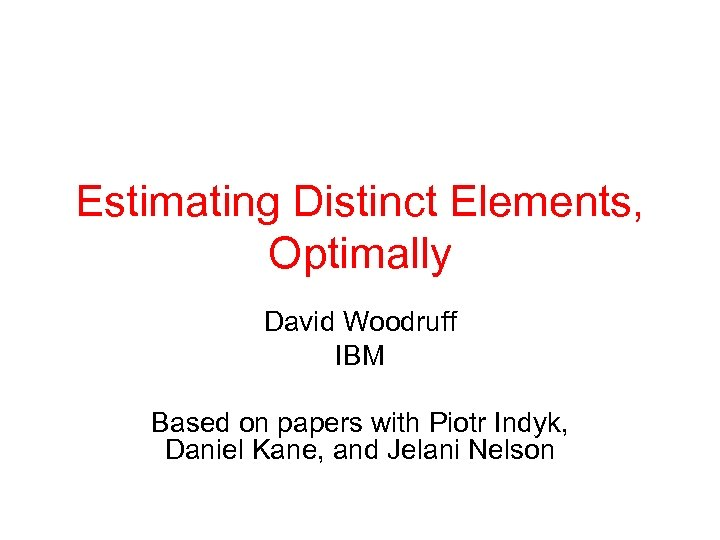Estimating Distinct Elements, Optimally David Woodruff IBM Based on papers with Piotr Indyk, Daniel Kane, and Jelani Nelson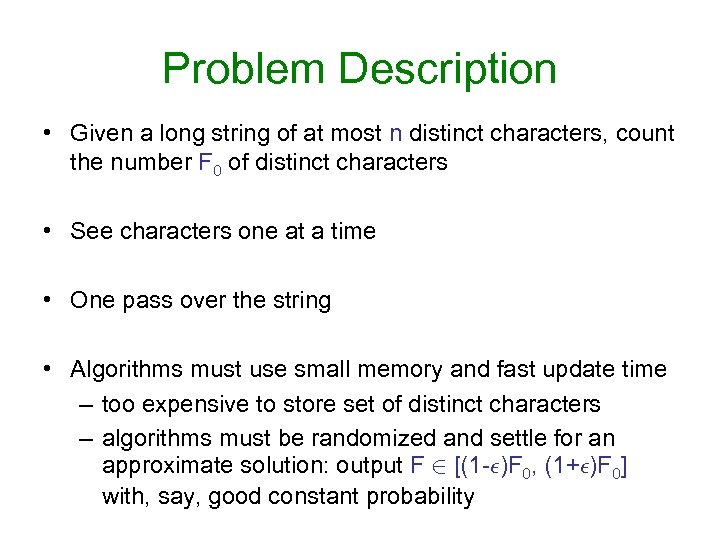Problem Description • Given a long string of at most n distinct characters, count the number F 0 of distinct characters • See characters one at a time • One pass over the string • Algorithms must use small memory and fast update time – too expensive to store set of distinct characters – algorithms must be randomized and settle for an approximate solution: output F 2 [(1 -²)F 0, (1+²)F 0] with, say, good constant probability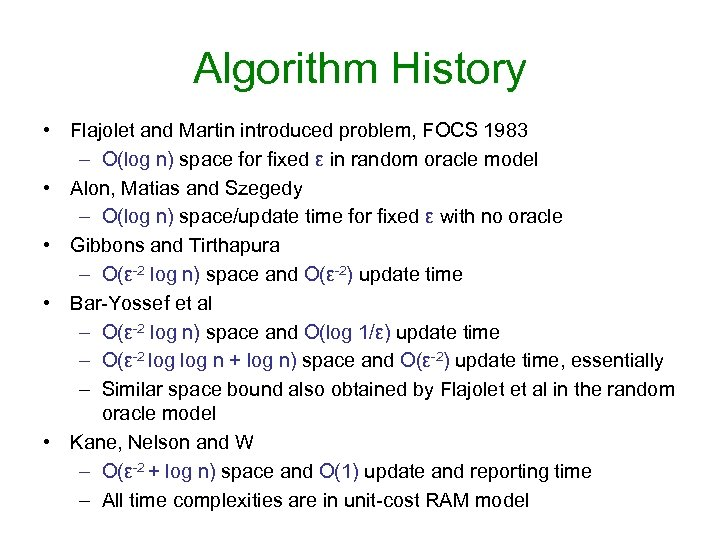Algorithm History • Flajolet and Martin introduced problem, FOCS 1983 – O(log n) space for fixed ε in random oracle model • Alon, Matias and Szegedy – O(log n) space/update time for fixed ε with no oracle • Gibbons and Tirthapura – O(ε-2 log n) space and O(ε-2) update time • Bar-Yossef et al – O(ε-2 log n) space and O(log 1/ε) update time – O(ε-2 log n + log n) space and O(ε-2) update time, essentially – Similar space bound also obtained by Flajolet et al in the random oracle model • Kane, Nelson and W – O(ε-2 + log n) space and O(1) update and reporting time – All time complexities are in unit-cost RAM model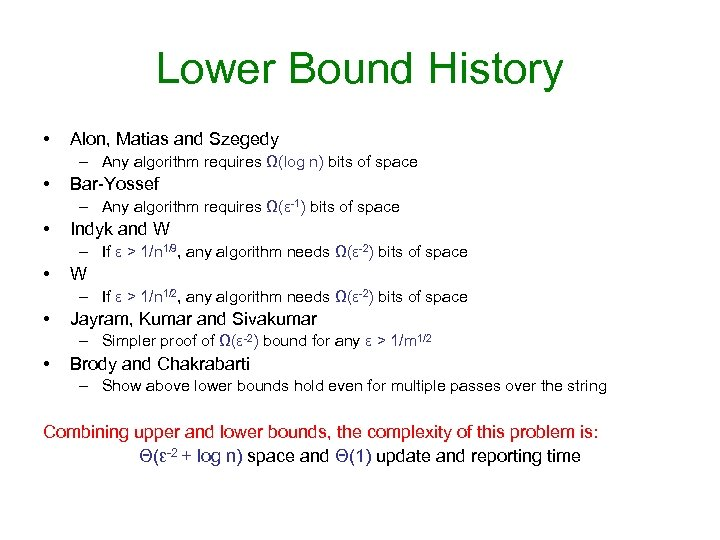Lower Bound History • Alon, Matias and Szegedy – Any algorithm requires Ω(log n) bits of space • Bar-Yossef – Any algorithm requires Ω(ε-1) bits of space • Indyk and W – If ε > 1/n 1/9, any algorithm needs Ω(ε-2) bits of space • W – If ε > 1/n 1/2, any algorithm needs Ω(ε-2) bits of space • Jayram, Kumar and Sivakumar – Simpler proof of Ω(ε-2) bound for any ε > 1/m 1/2 • Brody and Chakrabarti – Show above lower bounds hold even for multiple passes over the string Combining upper and lower bounds, the complexity of this problem is: Θ(ε-2 + log n) space and Θ(1) update and reporting time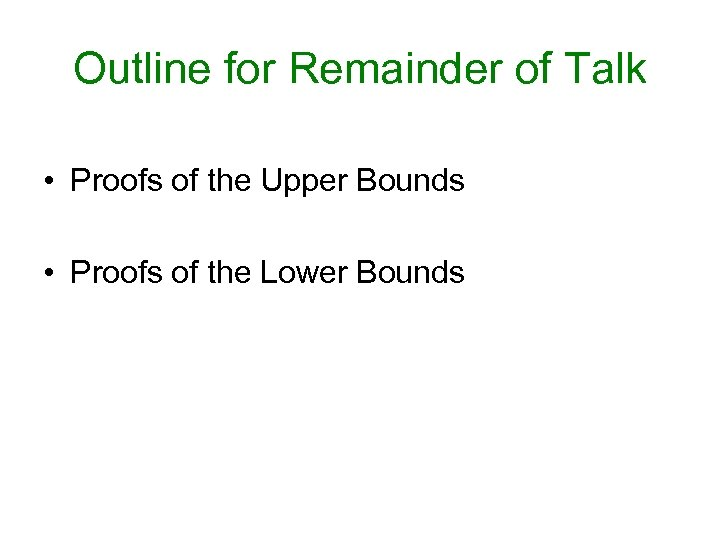Outline for Remainder of Talk • Proofs of the Upper Bounds • Proofs of the Lower Bounds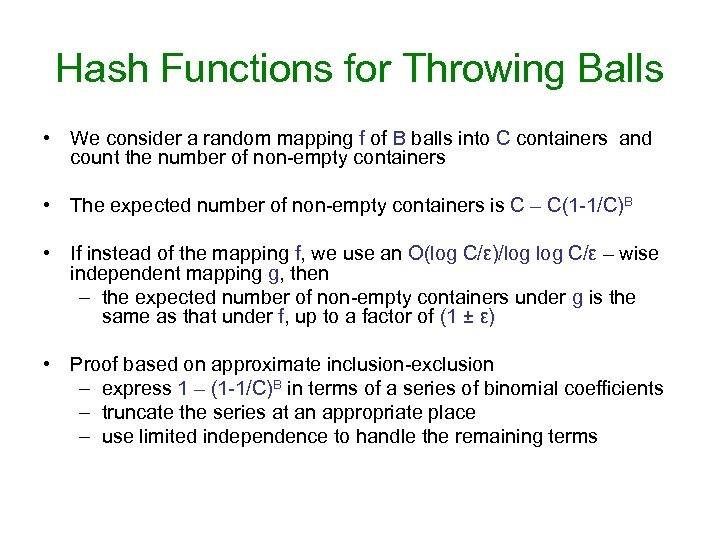Hash Functions for Throwing Balls • We consider a random mapping f of B balls into C containers and count the number of non-empty containers • The expected number of non-empty containers is C – C(1 -1/C)B • If instead of the mapping f, we use an O(log C/ε)/log C/ε – wise independent mapping g, then – the expected number of non-empty containers under g is the same as that under f, up to a factor of (1 ± ε) • Proof based on approximate inclusion-exclusion – express 1 – (1 -1/C)B in terms of a series of binomial coefficients – truncate the series at an appropriate place – use limited independence to handle the remaining terms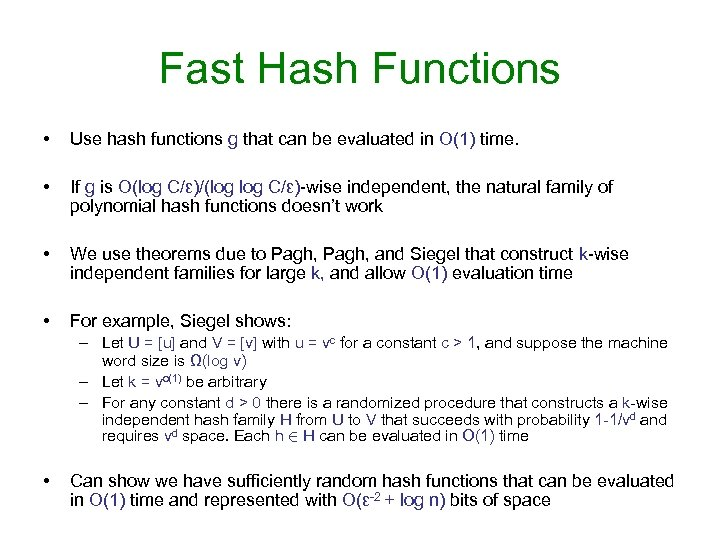Fast Hash Functions • Use hash functions g that can be evaluated in O(1) time. • If g is O(log C/ε)/(log C/ε)-wise independent, the natural family of polynomial hash functions doesn’t work • We use theorems due to Pagh, and Siegel that construct k-wise independent families for large k, and allow O(1) evaluation time • For example, Siegel shows: – Let U = [u] and V = [v] with u = vc for a constant c > 1, and suppose the machine word size is Ω(log v) – Let k = vo(1) be arbitrary – For any constant d > 0 there is a randomized procedure that constructs a k-wise independent hash family H from U to V that succeeds with probability 1 -1/vd and requires vd space. Each h 2 H can be evaluated in O(1) time • Can show we have sufficiently random hash functions that can be evaluated in O(1) time and represented with O(ε-2 + log n) bits of space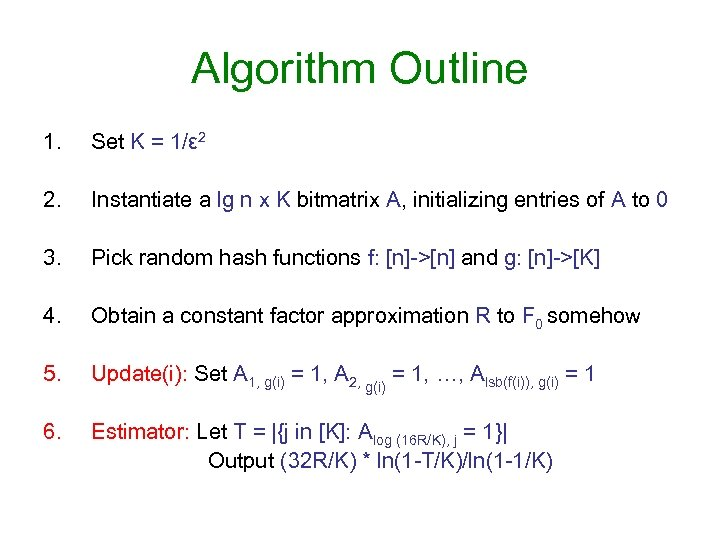Algorithm Outline 1. Set K = 1/ε 2 2. Instantiate a lg n x K bitmatrix A, initializing entries of A to 0 3. Pick random hash functions f: [n]->[n] and g: [n]->[K] 4. Obtain a constant factor approximation R to F 0 somehow 5. Update(i): Set A 1, g(i) = 1, A 2, g(i) = 1, …, Alsb(f(i)), g(i) = 1 6. Estimator: Let T = |{j in [K]: Alog (16 R/K), j = 1}| Output (32 R/K) * ln(1 -T/K)/ln(1 -1/K)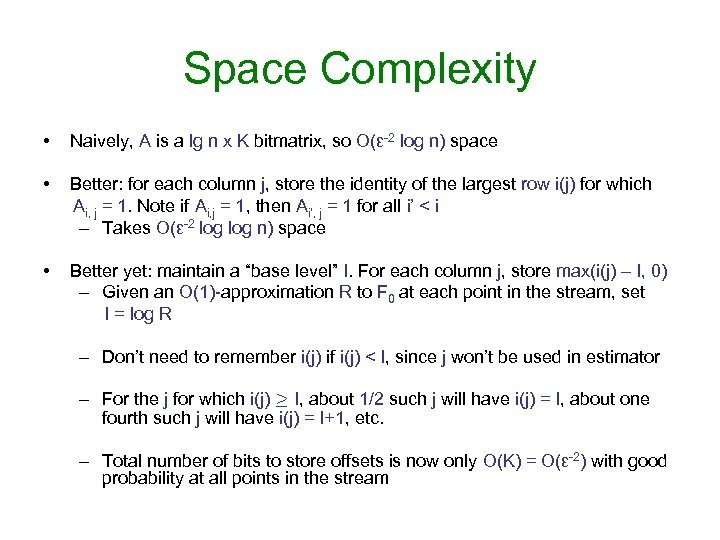Space Complexity • Naively, A is a lg n x K bitmatrix, so O(ε-2 log n) space • Better: for each column j, store the identity of the largest row i(j) for which Ai, j = 1. Note if Ai, j = 1, then Ai’, j = 1 for all i’ < i – Takes O(ε-2 log n) space • Better yet: maintain a “base level” I. For each column j, store max(i(j) – I, 0) – Given an O(1)-approximation R to F 0 at each point in the stream, set I = log R – Don’t need to remember i(j) if i(j) < I, since j won’t be used in estimator – For the j for which i(j) ¸ I, about 1/2 such j will have i(j) = I, about one fourth such j will have i(j) = I+1, etc. – Total number of bits to store offsets is now only O(K) = O(ε-2) with good probability at all points in the stream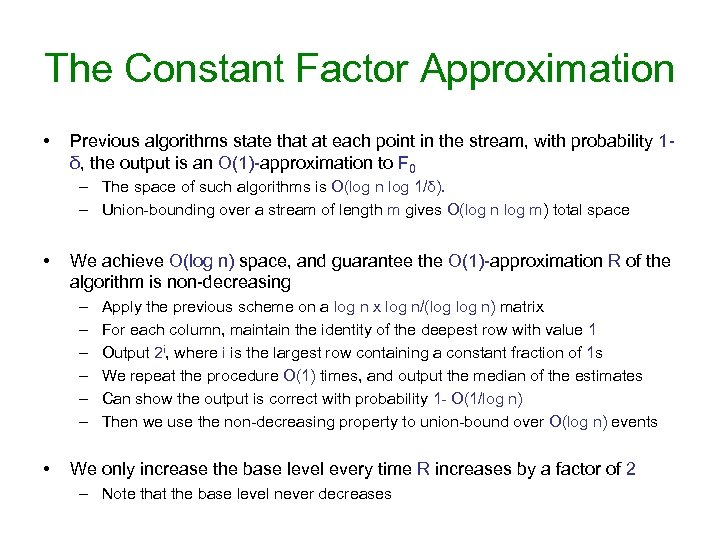The Constant Factor Approximation • Previous algorithms state that at each point in the stream, with probability 1δ, the output is an O(1)-approximation to F 0 – The space of such algorithms is O(log n log 1/δ). – Union-bounding over a stream of length m gives O(log n log m) total space • We achieve O(log n) space, and guarantee the O(1)-approximation R of the algorithm is non-decreasing – – – • Apply the previous scheme on a log n x log n/(log n) matrix For each column, maintain the identity of the deepest row with value 1 Output 2 i, where i is the largest row containing a constant fraction of 1 s We repeat the procedure O(1) times, and output the median of the estimates Can show the output is correct with probability 1 - O(1/log n) Then we use the non-decreasing property to union-bound over O(log n) events We only increase the base level every time R increases by a factor of 2 – Note that the base level never decreases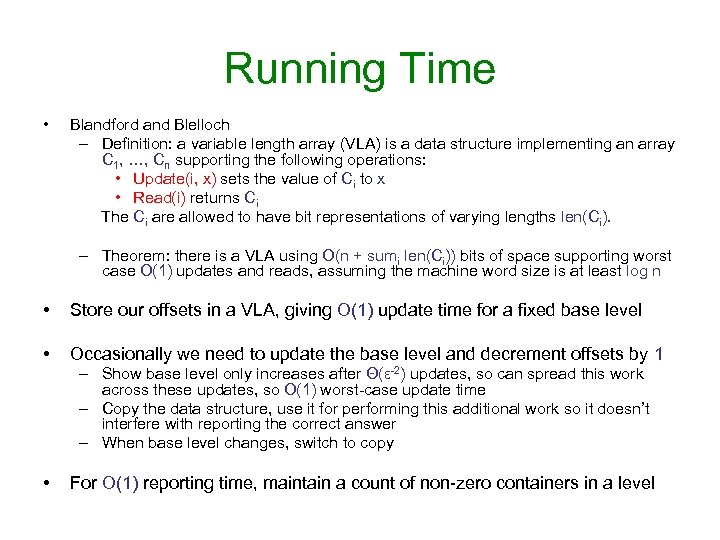Running Time • Blandford and Blelloch – Definition: a variable length array (VLA) is a data structure implementing an array C 1, …, Cn supporting the following operations: • Update(i, x) sets the value of Ci to x • Read(i) returns Ci The Ci are allowed to have bit representations of varying lengths len(Ci). – Theorem: there is a VLA using O(n + sumi len(Ci)) bits of space supporting worst case O(1) updates and reads, assuming the machine word size is at least log n • Store our offsets in a VLA, giving O(1) update time for a fixed base level • Occasionally we need to update the base level and decrement offsets by 1 – Show base level only increases after Θ(ε-2) updates, so can spread this work across these updates, so O(1) worst-case update time – Copy the data structure, use it for performing this additional work so it doesn’t interfere with reporting the correct answer – When base level changes, switch to copy • For O(1) reporting time, maintain a count of non-zero containers in a levelOutline for Remainder of Talk • Proofs of the Upper Bounds • Proofs of the Lower Bounds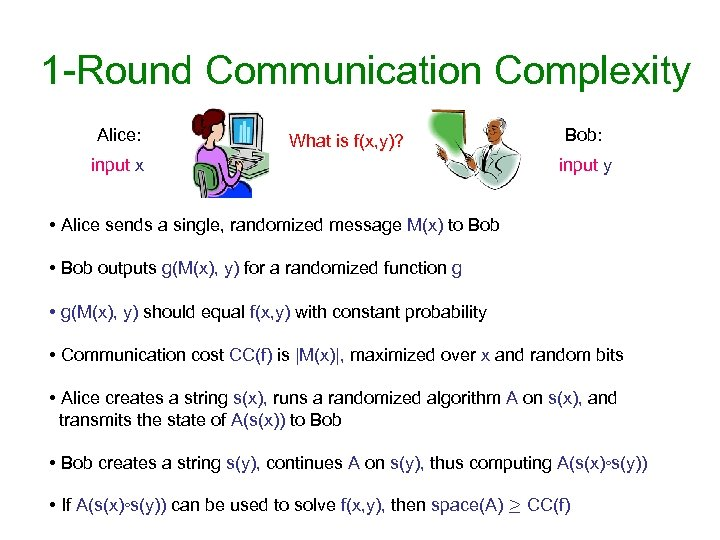1 -Round Communication Complexity Alice: What is f(x, y)? input x Bob: input y • Alice sends a single, randomized message M(x) to Bob • Bob outputs g(M(x), y) for a randomized function g • g(M(x), y) should equal f(x, y) with constant probability • Communication cost CC(f) is |M(x)|, maximized over x and random bits • Alice creates a string s(x), runs a randomized algorithm A on s(x), and transmits the state of A(s(x)) to Bob • Bob creates a string s(y), continues A on s(y), thus computing A(s(x)◦s(y)) • If A(s(x)◦s(y)) can be used to solve f(x, y), then space(A) ¸ CC(f)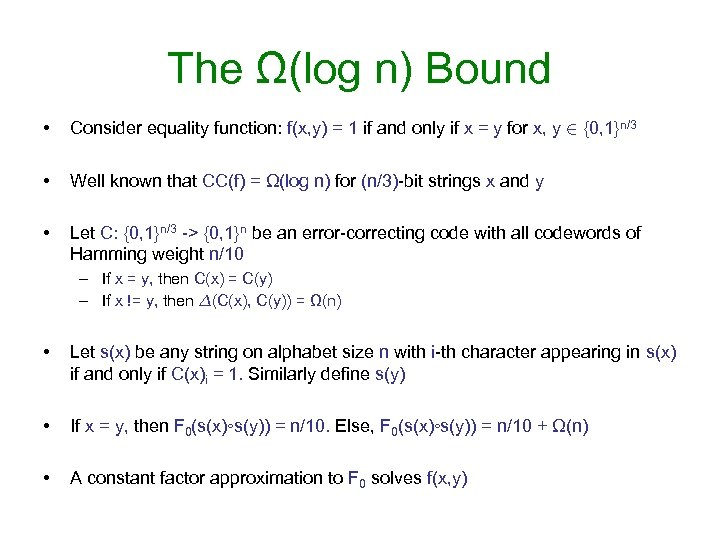The Ω(log n) Bound • Consider equality function: f(x, y) = 1 if and only if x = y for x, y 2 {0, 1}n/3 • Well known that CC(f) = Ω(log n) for (n/3)-bit strings x and y • Let C: {0, 1}n/3 -> {0, 1}n be an error-correcting code with all codewords of Hamming weight n/10 – If x = y, then C(x) = C(y) – If x != y, then ¢(C(x), C(y)) = Ω(n) • Let s(x) be any string on alphabet size n with i-th character appearing in s(x) if and only if C(x)i = 1. Similarly define s(y) • If x = y, then F 0(s(x)◦s(y)) = n/10. Else, F 0(s(x)◦s(y)) = n/10 + Ω(n) • A constant factor approximation to F 0 solves f(x, y)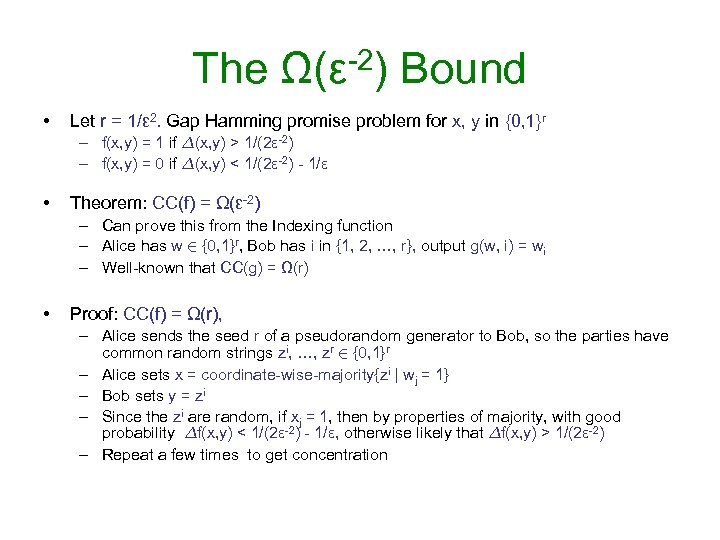The Ω(ε-2) Bound • Let r = 1/ε 2. Gap Hamming promise problem for x, y in {0, 1}r – f(x, y) = 1 if ¢(x, y) > 1/(2ε-2) – f(x, y) = 0 if ¢(x, y) < 1/(2ε-2) - 1/ε • Theorem: CC(f) = Ω(ε-2) – Can prove this from the Indexing function – Alice has w 2 {0, 1}r, Bob has i in {1, 2, …, r}, output g(w, i) = wi – Well-known that CC(g) = Ω(r) • Proof: CC(f) = Ω(r), – Alice sends the seed r of a pseudorandom generator to Bob, so the parties have common random strings zi, …, zr 2 {0, 1}r – Alice sets x = coordinate-wise-majority{zi | wj = 1} – Bob sets y = zi – Since the zi are random, if xj = 1, then by properties of majority, with good probability ¢f(x, y) < 1/(2ε-2) - 1/ε, otherwise likely that ¢f(x, y) > 1/(2ε-2) – Repeat a few times to get concentration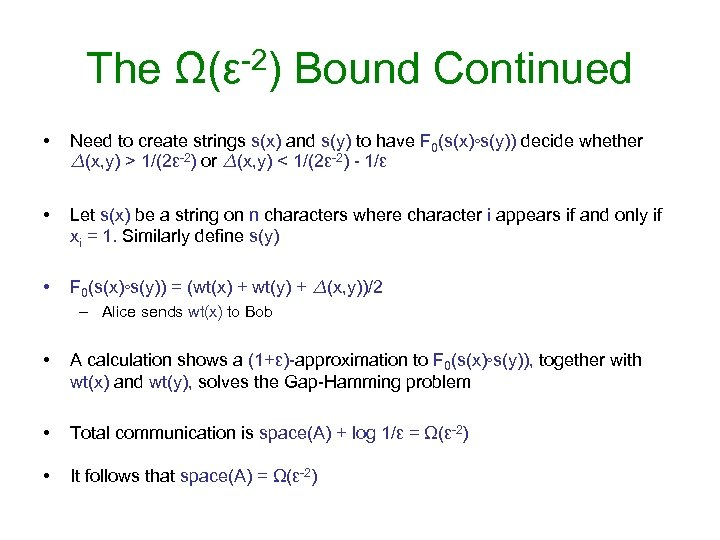The Ω(ε-2) Bound Continued • Need to create strings s(x) and s(y) to have F 0(s(x)◦s(y)) decide whether ¢(x, y) > 1/(2ε-2) or ¢(x, y) < 1/(2ε-2) - 1/ε • Let s(x) be a string on n characters where character i appears if and only if xi = 1. Similarly define s(y) • F 0(s(x)◦s(y)) = (wt(x) + wt(y) + ¢(x, y))/2 – Alice sends wt(x) to Bob • A calculation shows a (1+ε)-approximation to F 0(s(x)◦s(y)), together with wt(x) and wt(y), solves the Gap-Hamming problem • Total communication is space(A) + log 1/ε = Ω(ε-2) • It follows that space(A) = Ω(ε-2)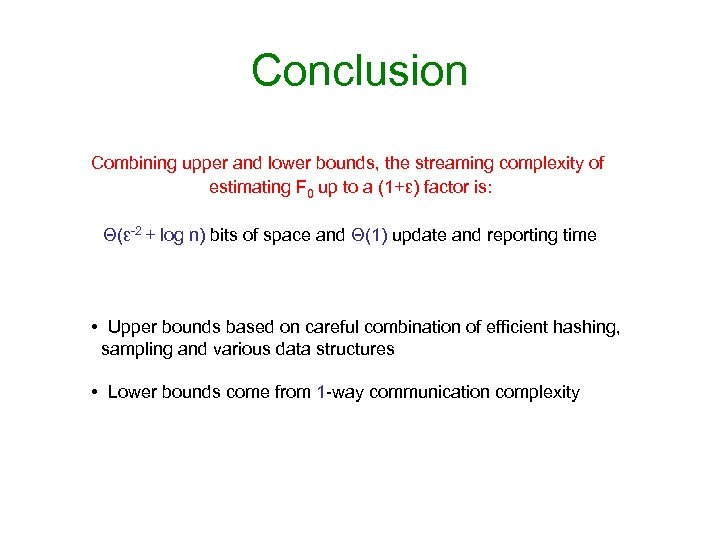Conclusion Combining upper and lower bounds, the streaming complexity of estimating F 0 up to a (1+ε) factor is: Θ(ε-2 + log n) bits of space and Θ(1) update and reporting time • Upper bounds based on careful combination of efficient hashing, sampling and various data structures • Lower bounds come from 1 -way communication complexity